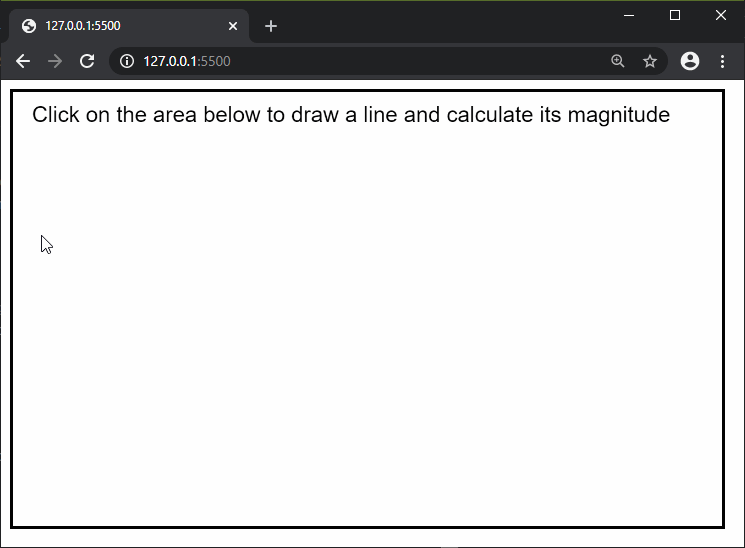# p5.js | mag() Function

The mag() function in p5.js is used to find the magnitude or length of a vector. As a vector does not have a starting position, the magnitude is calculated from position (0, 0) to (x, y). It is equivalent to using dist(0, 0, x, y).

Syntax:

`mag( a, b )`

Parameters: This function accept two parameters as mentioned above and described below:

• a: It is a number which denotes the first value, that is the “x” coordinate of the vector.
• b: It is a number which denotes the second value, that is the “y” coordinate of the vector.

Return Value: It returns a number with the magnitude of the vector.

Below examples illustrate the mag() function in p5.js:

Example:

 `function` `setup() { ` `  ``createCanvas(650, 400); ` `  ``strokeWeight(5); ` `  ``rect(0, 0, width, height); ` `  ``textSize(20); ` ` `  `  ``text(``"Click on the area below to draw"` `          ``+ ``" a line and calculate its "` `          ``+ ``"magnitude"``, 20, 30); ` `} ` ` `  `function` `mousePressed() { ` `  ``strokeWeight(1); ` ` `  `  ``// Draw line to where the ` `  ``// mouse is clicked ` `  ``line(0, 0, mouseX, mouseY); ` ` `  `  ``// Calculate the line magnitude ` `  ``lineMag = mag(mouseX, mouseY); ` ` `  `  ``// Draw the magnitude text on ` `  ``// the end of the line ` `  ``text(lineMag, mouseX, mouseY); ` `} `

Output:Online editor: https://editor.p5js.org/

Reference: https://p5js.org/reference/#/p5/magMy Personal Notes arrow_drop_upCheck out this Author's contributed articles.

If you like GeeksforGeeks and would like to contribute, you can also write an article using contribute.geeksforgeeks.org or mail your article to contribute@geeksforgeeks.org. See your article appearing on the GeeksforGeeks main page and help other Geeks.

Please Improve this article if you find anything incorrect by clicking on the "Improve Article" button below.

Article Tags :

Be the First to upvote.

Please write to us at contribute@geeksforgeeks.org to report any issue with the above content.